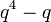# Linear representation theory of maximal unipotent subgroup of symplectic group over a finite field

This article gives specific information, namely, linear representation theory, about a family of groups, namely: maximal unipotent subgroup of symplectic group. This article restricts attention to the case where the underlying ring is a finite field.
View linear representation theory of group families | View other specific information about maximal unipotent subgroup of symplectic group | View other specific information about group families for rings of the type finite field

## Particular cases$m$$n = 2m$ maximal unipotent subgroup of symplectic group of degree$n = 2m$ Linear representation theory page for such a group over a finite field
1 2 additive group of the field, which, for a finite field, is an elementary abelian group --
2 4 maximal unipotent subgroup of symplectic group of degree four linear representation theory of maximal unipotent subgroup of symplectic group of degree four over a finite field
3 6 maximal unipotent subgroup of symplectic group of degree six linear representation theory of maximal unipotent subgroup of symplectic group of degree six over a finite field

## Degrees of irreducible representations

FACTS TO CHECK AGAINST FOR DEGREES OF IRREDUCIBLE REPRESENTATIONS OVER SPLITTING FIELD:
Divisibility facts: degree of irreducible representation divides group order | degree of irreducible representation divides index of abelian normal subgroup
Size bounds: order of inner automorphism group bounds square of degree of irreducible representation| degree of irreducible representation is bounded by index of abelian subgroup| maximum degree of irreducible representation of group is less than or equal to product of maximum degree of irreducible representation of subgroup and index of subgroup
Cumulative facts: sum of squares of degrees of irreducible representations equals order of group | number of irreducible representations equals number of conjugacy classes | number of one-dimensional representations equals order of abelianization

### Listing of degrees

We denote the field size by$q$ and the underlying prime by$p$. Let$r = \log_p q$.

First we consider the case where$p \ne 2$, so$q$ is odd.$m$$n = 2m$ Total number of irreps equals number of conjugacy classes in maximal unipotent subgroup of$Sp(n,q)$ Number of irreps of degree 1 Number of irreps of degree$q$ Number of irreps of degree$q^2$ Number of irreps of degree$q^3$
1 2$q$$q$
2 4$2q^2 - 1$$q^2$$q^2 - 1$
equals$(q - 1)(q + 1)$
3 6$q^4 + 4q^3 - 2q^2 - 3q + 1$$q^3$$q^4 - q$
equals$q(q - 1)(q^2 + q + 1)$$2q^3 - 2q^2$
equals$2q^2(q - 1)$$q^3 - 2q + 1$
equals$(q - 1)(q^2 + q - 1)$

### Partial sum values of squares of degrees

First we consider the case where$p \ne 2$, so$q$ is odd.$m$$n = 2m$ Order of maximal unipotent subgroup of$Sp(n,q)$ equals$q^{m^2} = q^{n^2/4}$ Sum of squares of degrees for degree 1 irreps Sum of squares of degrees for irreps of degree dividing$q$ Sum of squares of degrees for irreps of degree dividing$q^2$ Sum of squares of degrees for irreps of degree dividing$q^3$
1 2$q$$q$
2 4$q^4$$q^2$$q^4$
3 6$q^9$$q^3$$q^6$$2q^7 - q^6$$q^9$